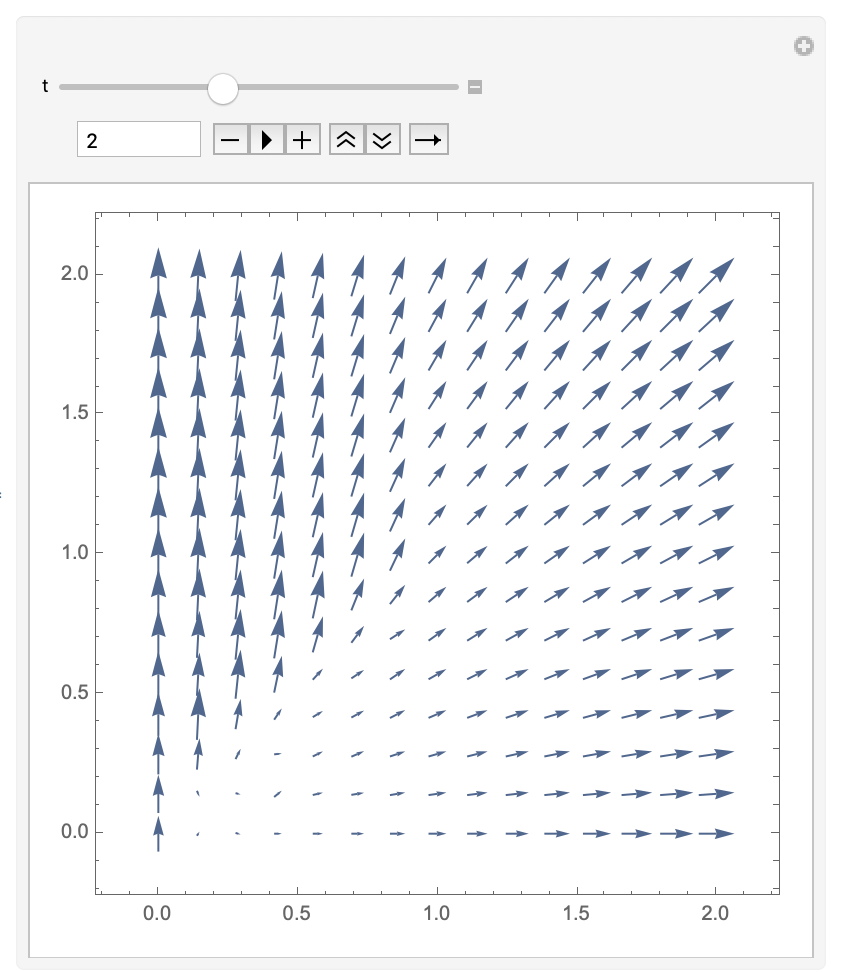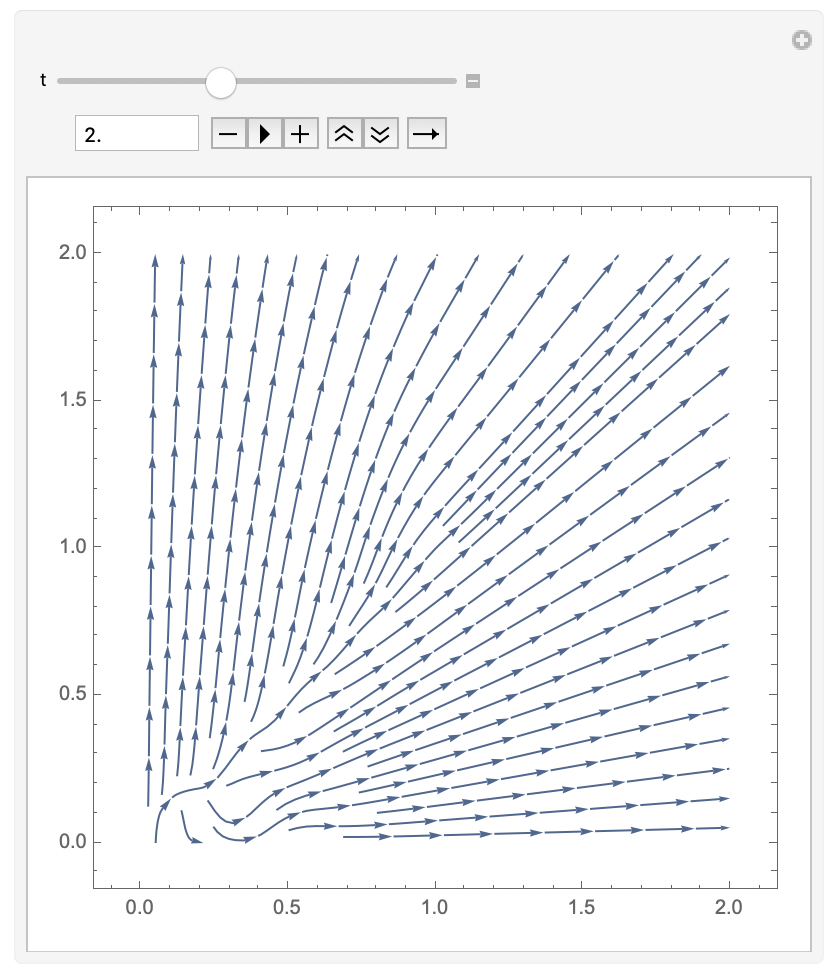# Solution of ODE with discontinuity

Let $$F:\mathbb{R} \to \mathbb R$$ be a bounded Lipschitz function and $$G(x,y) = (0,\chi_{\{x \le F(y)\}})$$.

Consider the ODE $$\begin{cases} \partial_t \Phi(t,x) = G(\Phi), & t \in [0,T]\\ \Phi(0,x) = x & x \in \mathbb R^2 \end{cases}$$

• How can we write the solution $$\Phi$$ explicitly?
• BTW, this is a PDE, not an ODE. Please fix it in the question.. Apr 25, 2019 at 4:06
• Also $G$ has two arguments, but you wrote $G(\Phi)$. Apr 25, 2019 at 4:20
• @user64494 $\Phi:\mathbb R^2 \to \mathbb R^2$. We use $\Phi_1$ as $x$ and $\Phi_2$ as $y$.
– Riku
Apr 25, 2019 at 11:48
• this still makes no sense; if $\Phi=(\Phi_1,\Phi_2)\in\mathbb{R}^2$, then $\Phi_2\in\mathbb{R}$; but in the definition of $G(x,y)$ you use $F(y)$ and if as you say $y$ should be replaced by $\Phi_2$, then you wish to evaluate the function $F$ at a point in $\mathbb{R}$, however, you have defined $F:\mathbb{R}^2\rightarrow\mathbb{R}$. Apr 25, 2019 at 11:54
• @CarloBeenakker That is a typo. Thank you. I'll edit it.
– Riku
Apr 25, 2019 at 11:55

OK, from the comments I understand that the problem boils down to

$$\frac{\partial}{\partial t}\Phi_2(t,x_1,x_2)=\theta\bigl(F[\Phi_2(t,x_1,x_2)]-x_1\bigr),\;\;\Phi_2(0,x_1,x_2)=x_2,$$

where $$\theta$$ is the unit step function. Let me assume that $$F$$ is a nondecreasing function. Then the solution is

$$\Phi_2(t,x_1,x_2)=\begin{cases} t+x_2&\text{if}\;\;F(x_2)>x_1\\ x_2&\text{if}\;\;F(x_2)\leq x_1. \end{cases}$$

A more general choice of $$F$$ can be readily accommodated, by piecing together increasing and decreasing segments. For any $$F$$, the function $$\Phi_2(t,x_1,x_2)=x_2$$ whenever $$F(x_2)\leq x_1$$, so we only need to consider regions in which $$F(x_2)>x_1$$ and $$\Phi_2$$ increases linearly with $$t$$ until $$F(\Phi_2)$$ becomes smaller than $$x_1$$.

as requested, the Mathematica code

Manipulate[Module[{sol = NDSolve[{phi2'[t] == UnitStep[Sin[phi2[t]] - x1], phi2 == x2}, phi2, {t, 0, tfinal}]}, Plot[Evaluate[phi2[t] /. sol], {t, 0, tfinal}]], {x1, 0, 2}, {x2, 0, 2}, {tfinal, 1, 10}]

will produce a plot of $$\Phi_2(t)$$ (for $$F=\sin$$) where you can vary $$t_{\rm final}$$, $$x_1$$, and $$x_2$$.

alternatively, the Mathematica code

sol = NDSolve[{D[phi2[t, x1, x2], t] == UnitStep[Sin[phi2[t, x1, x2]] - x1], phi2[0, x1, x2] == x2}, phi2, {t, 0, 5}, {x1, 0, 2}, {x2, 0, 2}]; Manipulate[VectorPlot[{x1, Evaluate[phi2[t, x1, x2] /. sol]}, {x1, 0, 2}, {x2, 0, 2}], {t, 0, 5}] Manipulate[StreamPlot[{x1, Evaluate[phi2[t, x1, x2] /. sol]}, {x1, 0, 2}, {x2, 0, 2}], {t, 0, 5}] 

will produce a vector plot or a stream plot of $$(\Phi_1,\Phi_2)$$ in the $$x$$-$$y$$ plane, with $$t$$ as a parameter that you can vary.A remark, too long for a comment. Let use the notation $$H=\mathbf 1_{\mathbb R_+}$$. You have to deal with an ODE whose flux is $$a(x,y)=H(F(y)-x),$$ where $$F$$ is a Lipschitz-continuous function, $$x$$ is a parameter. We have also formally $$\frac{\partial a}{\partial y}=\delta_0(F(y)-x)F'(y),$$ and assuming as in the previous answer that $$F$$ is monotone (say increasing), the rhs qualifies as the line-measure on the manifold with equation $$F(y)=x$$. As a result the flux is $$BV$$. Although there is a good theory for flow of $$BV$$ vector fields (say with bounded divergence), the second picture in the previous answer seems to indicate a non-uniqueness phenomenon, maybe related to the fact that the divergence of the vector field is unbounded.